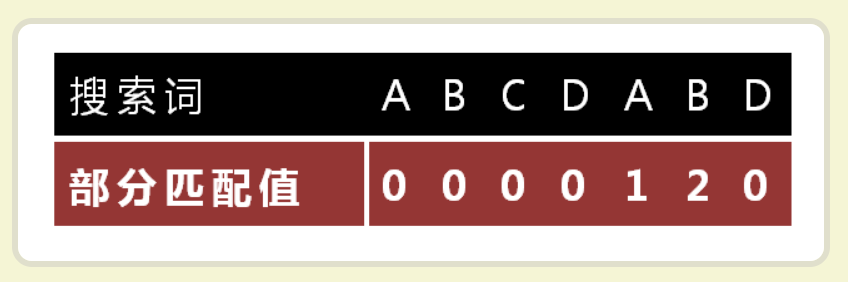# KMP（Java实现）

## 前缀和后缀的定义

• “前缀”指除了最后一个字符以外一个字符串的全部头部组合；
• “后缀”指除了第一个字符以外，一个字符串的全部尾部组合。
• 举例：

## 部分匹配值的定义

• “部分匹配值”就是”前缀”和”后缀”的最长的共有元素的长度
• 举例：字符串“”ABCDA””
• ”A”的前缀和后缀都为空集，共有元素的长度为0；
• “AB”的前缀为[A]，后缀为[B]，共有元素的长度为0；
• “ABC”的前缀为[A, AB]，后缀为[BC, C]，共有元素的长度0；
• “ABCD”的前缀为[A, AB, ABC]，后缀为[BCD, CD, D]，共有元素的长度为0；
• “ABCDA”的前缀为[A, AB, ABC, ABCD]，后缀为[BCDA, CDA, DA, A]，共有元素为”A”，长度为1；
• “ABCDAB”的前缀为[A, AB, ABC, ABCD, ABCDA]，后缀为[BCDAB, CDAB, DAB, AB, B]，共有元素为”AB”，长度为2；
• “ABCDABD”的前缀为[A, AB, ABC, ABCD, ABCDA, ABCDAB]，后缀为[BCDABD, CDABD, DABD, ABD, BD, D]，共有元素的长度为0。

## 定义一个方法用来计算一个字符串的部分匹配值

/**
* 根据 subStr 字符串 来计算出对应的部分匹配值
*
* @param subStr
* @return
*/
private static int calcMatchValue(String subStr) {

int length = subStr.length();
String preFixStr = subStr.substring(0, length - 1);
String suffFixStr = subStr.substring(1);

while (preFixStr.length() > 0 && suffFixStr.length() > 0) {
if (preFixStr.equals(suffFixStr)) {
return preFixStr.length();
}

if (preFixStr.length() == 1 && suffFixStr.length() == 1) {
break;
}
preFixStr = preFixStr.substring(0, preFixStr.length() - 1);
suffFixStr = suffFixStr.substring(1, suffFixStr.length());
}

return 0;
}


## 部分匹配表的定义

• 部分匹配表就是由每个字符串的部分匹配值产生的
•## 定义一个方法用来计算一个字符串的部分匹配表

/**
* 根据 pattern 字符串 创造出对应的部分匹配表
*
* @param pattern
* @return
*/
private static int[] createPartialMatchTable(String pattern) {

int patternLen = pattern.length();
int[] matchTable = new int[patternLen];

int i = 0;
int matchValue = 0;
while (i < patternLen) {
if (i == 0) {
matchValue = 0;
} else {
matchValue = calcMatchValue(pattern.substring(0, i + 1));
}

matchTable[i] = matchValue;
i++;
}

return matchTable;
}


## 使用kmp算法计算一个字符串是否包含在领一个字符串中

/**
* 使用KMP算法计算出 pattern字符串是否在target字符串当中
*
* @param target
*            目标串
* @param pattern
*            模式串
*/
private static boolean kmp(String target, String pattern) {

int[] partialMatchTable = createPartialMatchTable(pattern);

char[] targetCharArr = target.toCharArray();
char[] patterncharArr = pattern.toCharArray();
int matchCharCounts = 0;// 记录下已经匹配的字符的个数

int i = 0, j = 0, moveCounts = 0;
while (i < targetCharArr.length) {

// 如果当前主串和子串的字符匹配上了 那么比较下一个字符是否匹配
if (targetCharArr[i] == patterncharArr[j]) {
matchCharCounts++;
i++;
j++;
}
// 如果子串的第一个元素都不和珠串的元素相等 那么就拿主串的下一个元素进行比较
else if (j == 0) {
i++;
}
// 如果子串不是在第一个元素的位置而是在其他位置进行了失配，那么进行移位操作
else {
// 移动位数 = 已匹配的字符数 - 对应的部分匹配值
// 对应匹配值 指的是最后一个字符的对应匹配值 j是失配的位置 所以这里是partialMatchTable[j - 1]
moveCounts = matchCharCounts - partialMatchTable[j - 1];
j = j - moveCounts;//移动模式串 往前移moveCounts 位
matchCharCounts = matchCharCounts - moveCounts;//修改匹配的字符个数，就是减去移动过的位数
}

// 如果匹配成功了 直接返回true了
if (j == patterncharArr.length) {
return true;
}

}
return false;

}